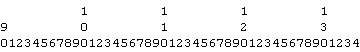Ben Nadel at cf.Objective() 2014 (Bloomington, MN) with: Jeff McDowell ( @jeff_s_mcdowell ) Joel Hill ( @Jiggidyuo ) Jonathan Rowny ( @jrowny ) Shawn Grigson ( @shawngrig ) Jonathan Dowdle ( @jdowdle ) Matt Vickers ( @envex ) Christian Ready ( @christianready ) Asher Snyder ( @ashyboy ) Clark Valberg ( @clarkvalberg ) Oscar Arevalo ( @oarevalo ) David Bainbridge ( @redwhitepine ) Josh Siok ( @siok )

# Creating An ASCII Ruler In ColdFusion

By on
Tags:

I posted this as a solution to CF-Talk the other day and I thought I would post it here as well (for some reason, not everyone and their mother subscribes to CF-Talk??). Paul Vernon wanted to create an on-screen ASCII ruler that would fully display the powers of 10 (10, 20, 30... 150, 160, etc) but for each non-power-of-10, only display the ones digit (1,2,3,4,5,67,8,9).

My solution builds the output into a two dimensional array. I was treating each character in the output as a sepparate index in the array. Places that has no digit were given a white space character. Then the array is converted to a string using a Java StringBuffer.

I am not 100% sure what this would ever be used for, but here goes:

``````<!--- Get the length of the ASCII ruler. --->
<cfset intRulerLength = 150 />

<!---
Get the ruler height. This is the number of digits that
the max value will have. Since the rule will count up from
1, the max height will be determined by the last value in
the ruler (ie. the length of the ruler).
--->
<cfset intRulerHeight = Len( intRulerLength ) />

<!---
Create the array in which to build the output. This is a
two-dimensional array. The first index is going to create
the ROW of the output (digit column). The Second index is
going to be the COLUMN of the output (the index on the ruler.
--->
<cfset arrRuler = ArrayNew( 2 ) />

<!---
Now, let's start building the ruler output by looping from
1 to the length of the ruler.
--->
<cfloop index="intColumn" from="1" to="#intRulerLength#">

<!---
Check to see if we are at a power of 10 (MOD will not
return a remainder). If we are, then we need to display
all digits, otherwise, we are just going to display the
ones digit column.
--->
<cfif (intColumn MOD 10)>

<!--- Fill the entire column with spaces. --->
<cfloop index="intDigit" from="1" to="#intRulerHeight#">

<cfset arrRuler[ intDigit ][ intColumn ] = " " />

</cfloop>

<!---
Now that we have filled the column with spaces, put
the ones digit in the last space.
--->
<cfset arrRuler[ intRulerHeight ][ intColumn ] = (intColumn MOD 10) />

<cfelse>

<!---
We need to output the entire value in the output.
But, this value might not be the same length as the
max value length. Therefore, we need to
right-justify the value to fit exactly with the
ruler's height.
--->
<cfset strValue = RJustify(
intColumn,
intRulerHeight
) />

<!---
Now loop over the value and apply to the ruler
output array.
--->
<cfloop index="intDigit" from="1" to="#intRulerHeight#">

<cfset arrRuler[ intDigit ][ intColumn ] = Mid(
strValue,
intDigit,
1
) />

</cfloop>

</cfif>

</cfloop>

<!---
ASSERT: At this point, the entire output for the ASCII ruler
should be stored in the ruler array. Now, we need to
translate that into a horizontal ruler. To make this faster
and control the white space, let's create a Java string
buffer to which we can systematically append output data.
--->
<cfset sbOutput = CreateObject(
"java",
"java.lang.StringBuffer"
).Init() />

<!---
Loop over the ruler array and append to output. Since we
need to output the ruler horizontally, we need to do each
row at a time.
--->
<cfloop index="intDigit" from="1" to="#intRulerHeight#">

<!--- For each row of digits, loop over every column. --->
<cfloop index="intColumn" from="1" to="#intRulerLength#">

<!---
When appending, we need to convert the array data
to a string to make sure Java don't choke.
--->
<cfset sbOutput.Append(
JavaCast(
"string",
arrRuler[ intDigit ][ intColumn ]
)
) />

</cfloop>

<!--- After each row, add a line break. --->
<cfset sbOutput.Append(
JavaCast(
"string",
Chr( 13 ) & Chr( 10 )
)
) />

</cfloop>

<!--- Output the ASCII data in PRE tags for best formatting. --->
<pre>#sbOutput.ToString()#</pre>
``````

This gives us output that looks like this:So, not sure what this is used for, but that's one solution.

Want to use code from this post? Check out the license.